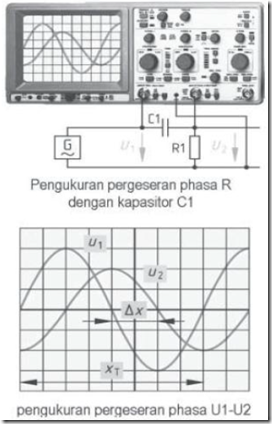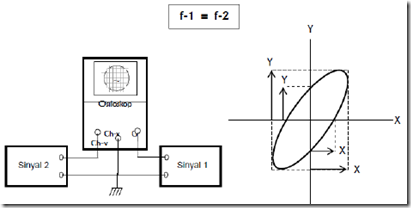Home > English > Phase Power Measurement

# Phase Power Measurement

A. Measuring the phase difference with Electric Current Voltage AC.
Phase difference can be measured by a series of C1 danR1. Voltage U1 appeared from the supply voltage AC generator. U2 voltage divided by the value of resistor R1 representation of an AC power outlet. U1 with U2 phase shifts of Dx.
For example: φ = Δx · 360 ° / XT
Div = 2 · 360 ° / 8div = 90 °
Display sinusoidal signal voltage U1 (power supply voltage) and the voltage U2 (when divided by R1, representation of the AC current).
Phase shift between the voltage and current of φ = 900Figure 9.6 Measurement Different Phases

B. Method of Lissajous
Two signals can be measured depending phasanya by utilizing vertical input (channels Y) and horizontal (canal-X). By using two-channel oscilloscope can show different phase known as Lissajous method.Figure 9.7 Principles of Measurement Method Lissajous
The phase difference of 0 ° or 360 °.

Two different signals, in this case the input signal and the output signal if combined would generate configuration entirely different shape. Input signal is fed to the channel Y (vertical) and the output signal is inserted into the channel X (horizontal) is different from 0 °, combined will produce alloy signal is a straight line that forms an angle of 45 °.Figure 9.8 Different Phases zero or 360o between Input and Output
The phase difference of 90 ° or 270 °.

Vertical signal sinusoidal signal form. Horizontal different signal phase 90 ° or 270 ° included. Alloys results that appear on the CRT screen is rounded lines.Figure 9.9 Different Phases 90 ° or 270 ° between Input and Output"Tasikmalaya, Indonesia"